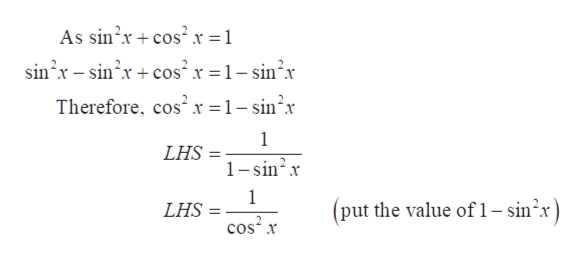Question
8 views

verify each identity:

1                 = 1 + tan2x

1-sin2x

check_circle

Step 1

First of all, consider the given identity to prove.

The given equation is an identity.

Step 2

Now, consider only the LHS.

Use trigonometric ide...help_outlineImage TranscriptioncloseAs sin?x + cos² x = 1 sin'x – sin?x + cos x =1- sin?x Therefore, cos'x =1- sin?x LHS 1-sin?x 1 (put the value of 1– sin’x) LHS cos x fullscreen

### Want to see the full answer?

See Solution

#### Want to see this answer and more?

Solutions are written by subject experts who are available 24/7. Questions are typically answered within 1 hour.*

See Solution
*Response times may vary by subject and question.
Tagged in

### Calculus